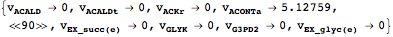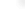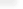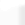Toolbox Tutorial

# Constraint-based modeling

The following sections provide a very general introduction into the constraint-based modeling capabilities of the toolbox. More detailed information can be obtained from the individual documentation pages of the respective commands. A primer and a review of constraint-based modeling can be found here or here.
Load a model of Escherichia coli central metabolism

### Constraints

Constraint-based modeling evaluates phenotypes in the light of biological, physical, and chemical constraints. One important type of constraints are flux capacities, e.g, a reaction is irrversible under physiological conditions and can thus carry only positive net-flux. Toolbox models provide a Constraints attribute where this kind of information can be included. Lower and upper bounds on reactions fluxes are encoded using rules of flux identifiers and pairs of
 vid→{lowerBound, upperBound} Single flux constraint getConstraints[model] or model["Constraints"] access the model constraints setConstraints[model, constr] overwrite previous constraints with new constraints (constr) updateConstraints[model, constr] udpate existing constraints with new constraints (constr)

Dealing with constraints

Query all available constraints.
 Out=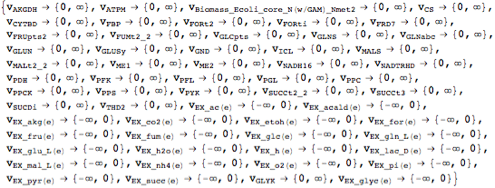Looking at the flux bounds of all availabe exchange reactions reveals that the current model can only infinitely secrete but not take up any matter.
 Out=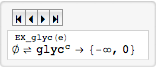Change a few exchange reaction flux bounds to reflect an aerobic minimal glucose medium.

### Flux-balance and flux-variability analysis

Flux-balance analysis (FBA) finds a steady-state flux solution that maxmizes a cellular objective, e.g., optimal growth rate. For example, FBA can be used to asses the consequence of genetic perturbations. Flux-variablity analysis (FVA) calculates effective flux bounds by minimizing and maximizing flux through individual reactions. For example, FBA solutions are rarely unique and FVA can be used to assess the space of alternativa optimal solutions.
 fba[model, obj] find a steady-state flux distribution that maximizes obj fva[model] find minimum and maximum fluxes for all reactions in model

Basic COBRA functionality

Perform FBA.
 Out=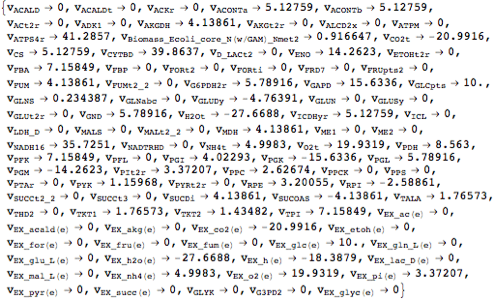Visualize the fluxes on a pathway map.
 Out=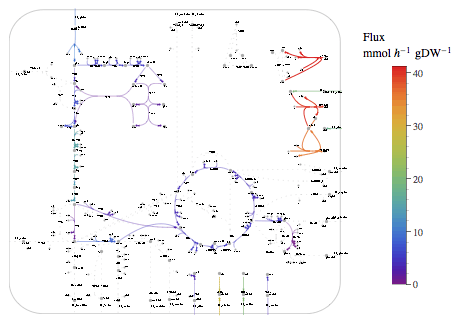Perform FVA.
 Out=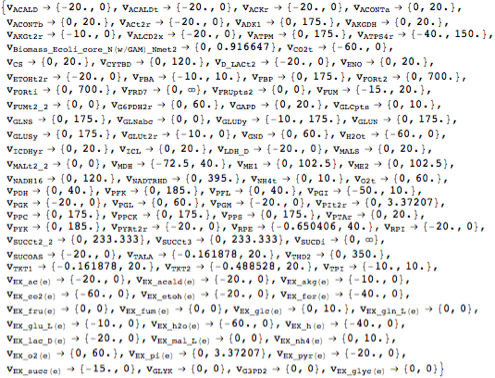Visualize FVA result.
 Out=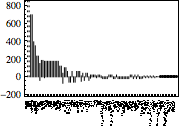### High-throughput data integration

In progress ...
 gimme[...] find a steady-state flux distribution that maximizes obj and matches data

XXXX.

Perform GIMME

### Changing linear programming solver back-ends

Per default, the toolbox uses Mathematica's built-in linerar programming solver (LinerarProgramming). Depending on the availability of other optimization tools, different solver back-ends can be used to solve constraint-based modeling problems.
 LinearProgramming Mathematica's internal linear programming solver GurobiSolve MathLink interface to Gurobi GLPKStandalone Interface to the GLPK standalone solver CPLEXStandalone Interface to the CPLEX standalone solver

Supported solver back-ends

 Out//Short=